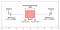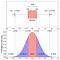# How to Handle Outliers in Machine Learning

Hello Everyone!!!! The most important phase in Feature Engineering is handling outliers because it ensures that our model is trained on accurate data which leads to accurate models.

Today we’ll look at what outliers are, their causes and consequences, various ways to identifying them, and finally various methods for dealing with them using code samples.

The code sample and dataset for this article are available here.

## What is an Outlier

A data point that varies greatly from other results is referred to as an outlier.

An outlier may also be described as an observation in our data that is incorrect or abnormal as compared to other observations.

Causes and Consequences

Outliers can be caused by measurement uncertainty or due to experimental error.

Outliers in data can spoil and deceive the training process of machine learning models, resulting in less accurate models and eventually bad performance.

Now that we know what outliers are and how they affect Machine Learning algorithms, let’s look at how we can detect them in our data.

How to detect Outliers

Outliers in data can be observed using a number of techniques. In this article, we’ll look at the most popular method, which is the visualization technique.

To find outliers, we can simply plot the box plot. Outliers are points that are outside of the minimum and maximum values, as seen in the image below.Blox-plot representation

How to Measure the Outliers

We can measure the boundary for outliers once we’ve decided whether outliers are present in the data using the box plot.
To measure the boundary for outliers, we can use the two methods below, both based on data distribution.

I) If the Data is Normally Distributed

We can use the empirical formula of Normal Distribution to determine the boundary for outliers if the data is normally distributed.

Lower Boundary = Mean — 3* (Standard Deviation)

Upper Boundary= Mean + 3 * (Standard Deviation)Normal Distribution of Box Plot with Standard Deviation

Let’s have a look at the below code to find the outliers boundaries for our dataset:

II) If the Data is Either Right Skewed or Left Skewed

We will use the Interquartile Range to measure the limits of Outliers if the data doesn’t follow a Normal Distribution or is either right-skewed or left-skewed.

Interquartile Range(IQR) = Q3(75th percentile) -Q1(25th percentile)

The formula for the outlier boundary can be calculated as:

Lower Boundary= First Quartile(Q1/25th percentile) — (1.5 * IQR)

Upper Boundary = Third Quartile(Q3/75th percentile) +(1.5* IQR)

If the outlier’s maximum value is extremely high in comparison to the upper boundary, the boundary of outliers (also known as extreme outliers) will be calculated using the formula below:

Lower Boundary= First Quartile(Q1/25th percentile) — (3 * IQR)

Upper Boundary = Third Quartile(Q3/75th percentile) +(3 * IQR)

Let’s have a look at the below code to find the outliers boundaries for Fare Column:

Following approaches can be used to deal with outliers once we’ve defined the boundaries for them:

1. Remove the observations
2. Imputation

1.Remove the Observations

We may explicitly delete outlier observation entries from our data so that they don’t influence the training of our models. When dealing with a small dataset, however, eliminating the observations is not a good idea.

2. Imputation

To impute the outliers, we can use a variety of imputation values, ensuring that no data is lost.
As impute values, we can choose between the mean, median, mode, and boundary values.

References

Congratulations on learning how to deal with outliers while doing Feature Engineering on the data.

Thank you for taking the time to read this post. If you liked this read, hit the 👏 button and share it with others. You can also check other interesting articles under my Medium profile. If any questions, please leave them in the comments section and I will do my best to answer them.

You can connect with me on LinkedIn, Facebook, and Instagram.

Until next time, Adios Amigo!!!!

## Analytics Vidhya

Analytics Vidhya is a community of Analytics and Data…

## Analytics Vidhya

Analytics Vidhya is a community of Analytics and Data Science professionals. We are building the next-gen data science ecosystem https://www.analyticsvidhya.com

Written by

## Ashutosh Sahu

Learning, Implementing and Sharing## Analytics Vidhya

Analytics Vidhya is a community of Analytics and Data Science professionals. We are building the next-gen data science ecosystem https://www.analyticsvidhya.com

## More From Medium

Medium is an open platform where 170 million readers come to find insightful and dynamic thinking. Here, expert and undiscovered voices alike dive into the heart of any topic and bring new ideas to the surface. Learn more

Follow the writers, publications, and topics that matter to you, and you’ll see them on your homepage and in your inbox. Explore

If you have a story to tell, knowledge to share, or a perspective to offer — welcome home. It’s easy and free to post your thinking on any topic. Write on Medium